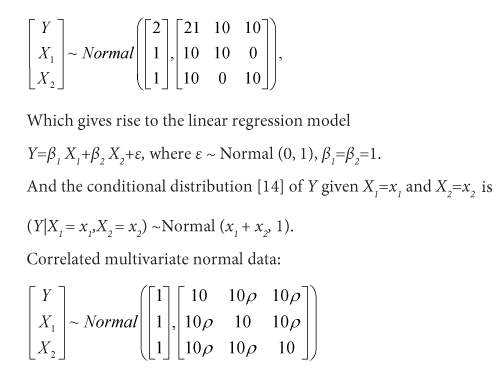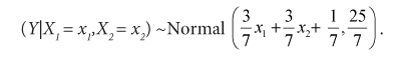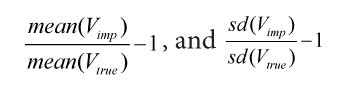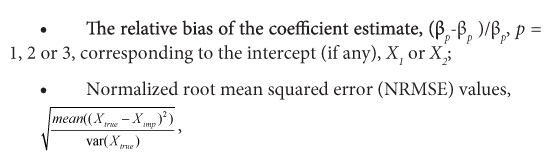Parallelization Using Different Strategies and its Influence on Random-Forest-Based Statistical Methods: A Case Study on Iterative Missing Data ImputationResearch Article

Austin Biom and Biostat. 2021; 6(1): 1037.

# A Note on the Required Sample Size of Model-Based Dose-Finding Methods for Molecularly Targeted Agents

Hong S, Sun Y, Li H and Lynn HS*

Department of Biostatistics, Fudan University, China

*Corresponding author: Lynn HS, Department of Biostatistics, School of Public Health, Key Laboratory on Public Health Safety of the Ministry of Education, Fudan University, Shanghai, China

Received: December 08, 2020; Accepted: February 24, 2021; Published: March 03, 2021

## Abstract

Random forest has proven to be a successful machine learning method, but it also can be time-consuming for handling large datasets, especially for doing iterative tasks. Machine learning iterative imputation methods have been well accepted by researchers for imputing missing data, but such methods can be more time-consuming than standard imputation methods. To overcome this drawback, different parallel computing strategies have been proposed but their impact on imputation results and subsequent statistical analyses are relatively unknown. Newly proposed random forest implementations, such as ranger and randomForestSRC, have provided alternatives for easier parallelization, but their validity for doing iterative imputation are still unclear. Using random-forest imputation algorithm missForest as an example, this study examines two parallelized methods using newly proposed random forest implementations in comparison with the two parallel strategies (variable-wise distributed computation and model-wise distributed computation) using language-level parallelization from the software package. Results from the simulation experiments showed that the parallel strategies could influence both the imputation process and the final imputation results differently. Different parallel strategies can improve computational speed to a variable extent, and based on simulations, ranger can provide performance boost for datasets of different sizes with reasonable accuracy. Specifically, even though different strategies can produce similar normalized root mean squared prediction errors, the variable-wise distributed strategy led to additional biases when estimating the mean and inter-correlation of the covariates and their regression coefficients. And parallelization by randomForestSRC can lead to changes in both prediction errors and estimates.

Keywords: Random forest; Parallel computation; Missing data iterative imputation

## Abbreviations

OOB: Out-of-Bag; RF: Random Forest; MCAR: Missing Completely at Random; MAR: Missing at Random

## Introduction

Random forest has proven to be a successful machine learning method with successful applications . As missing data are common in most research, various kinds of imputation methods have been proposed for handling missing data problems. Stekhoven and Buhlmann  proposed the missForest algorithm based on a Random Forest (RF) machine learning method , and it has been used in different studies and benchmarked against other imputation methods [4-7]. MissForest has been shown to have superior predictive accuracy under certain circumstances, but it necessitates the building of a large number (default is 100) of trees during the imputation process for a single variable per iteration and usually several iterations are required. Likewise, missForest can be computationally intensive and time-consuming for large datasets, thereby limiting its usability. Moreover, various multiple imputation methods have been proposed based on random forests , and they all do iterative computations.

To boost performance, two parallel computing strategies (referred to as “forests” and “variables” in the software package) suitable for “long” and “wide” datasets, respectively , were implemented in missForest with the release of version 1.4. However, there has not been any published evaluation of their difference on predictive accuracy and subsequent impact on statistical analyses. The implicit assumption is that these two strategies are equally valid and will lead to similar results. Recently, new implementations of the RF algorithm, like ranger  and randomForestSRC  software packages, have been proposed to provide new functionalities and computational speed improvements. But applications of such implementations in doing iterative prediction tasks are rarely discussed and their influences on the results are still unknown.

Using missForest algorithm as an example, the algorithm was reimplemented using the two recently proposed RF software packages, and this study uses simulation experiments to address the differences in both imputation accuracy and computation efficiency among these parallel computation strategies of missForest. Computational efficiency can be critical for handling large datasets; thus, this study’s results can be of use to both data analytics practitioners and methodologists for imputation methods.

## Materials and Methods

MissForest algorithm

In missForest, the variables containing missing values are initialized for imputation by replacing the missing cells by corresponding mean values (for continuous variables), or by the most frequent category (for categorical variables). A variable under imputation is then divided into two distinct parts: the observed part that contains no missing values, and the missing part that serves as the prediction set. A random forest is fitted using the observed part as response and the corresponding values of the other variables as predictors, and the missing part is replaced with the predicted values from the random forest. The algorithm then proceeds to the next variable to be imputed, and the iteration stops when the difference between the current and previously imputed values increase or if the maximum number iteration is reached. Since the release of missForest version 1.4, two parallel strategies have been implemented to increase the computational efficiency when applying random forest imputation to large datasets.

Strategy 1: distributing the computation of imputing a single variable: In the first strategy, the building of the ensemble of trees for a variable to be imputed are divided into smaller subsets and distributed into different computing processes based on the number of core processors in the computer. The results from different ensembles of smaller trees are recombined into a single one, and the final predictions are derived from the combined ensemble of trees. Each variable to be imputed undergoes this process until all the variables have been imputed in a single iteration. This strategy is most useful if the process of building a random forest is time-consuming and the number of variables in the dataset is relatively small.

Strategy 2: distributing the computation of different variables: In the second strategy, the computation of the random forest for each variable to be imputed in a single iteration is distributed to different computing processes. The imputations of the variables are done simultaneously and independent of each other with the building of the ensemble of trees for each variable performed by a single process. After all the variables have been imputed, the results are recombined to form a single complete dataset. The current iteration is then finished, and the algorithm moves to the next iteration. This strategy can be useful for datasets containing many variables while the time consumption for building the random forest for a single variable is small.

Accelerated random forests

The R software packages, ranger and randomForestSRC, have extended the original random forest algorithms in different ways and they both provided parallelized implementations of the random forest algorithm. The ranger software package is fast implementation of random forest, particularly suited for high dimensional data. And the “rfsrc.fast” function in randomForestSRC provides fast approximate random forests. Both software packages support classification, regression, and survival forests. The missForest algorithm was reimplemented using ranger and randomForestSRC with parameters adjusted to eliminate differences in sampling processes, as the “rfsrc.fast” function in randomForestSRC does not do bootstrap sampling by default. The source code for self-written software package used in this study can be found online .

Simulation studies

To further investigate the influence of the choice of parallel strategies on imputation, a series of simulations and analyses were carried out using R, version 3.6 (R Core Team, Vienna, Austria) . Four sequential stages were involved:

• Data generation: Complete datasets were simulated based on pre-defined scenarios.
• Amputation: The complete datasets were made incomplete based on specified rules.
• Imputation: the missing values contained in the simulated datasets were filled in by missForest using different parallel strategies.
• Analysis: Statistical analysis were performed on both the original complete datasets and the corresponding imputed datasets, and comparisons were made.

The computational time costs of different strategies were also compared based on simulations of missing completely at random (MCAR) data containing 50% missing data cells on a laptop computer with a multi-core CPU installed to demonstrate the performance gain using different parallel strategies.

Data generation: The data structures were made as simple as possible with a response Y and just two covariates X1 and X2 to enhance the investigation of the influence of the two parallel strategies on imputation results. Also, a large variance was used to get more discriminative results. Three different sets of 2000 simulated datasets containing 200 observations each were generated based on following settings:

Uncorrelated covariates with linearly dependent response:The correlation coefficients were ρ=0.25 or ρ=0.75, roughly corresponding to weakly correlated and strongly correlated data. This multivariate distribution leads to the following conditional distributions of Y given X1 = x1 and X2 = x2:

(Y|X1 = x1,X2 = x2, X2=x2) ~Normal (0.2x1+0.2x2+0.6, 9), andAltogether, the simulation consists of three different data generation scenarios: (1) multivariate normal data with independent covariates; (2) moderately correlated multivariate normal data; and (3) strongly correlated multivariate normal data.

Amputation: Amputation functions  provided by the “MICE”  R package were used in this study to generate missing values. Missing at random (MAR) patterns were introduced by setting X1 and/or X2 to be missing depending on Y. Specifically, the probability of each observation being missing was set to 50% according to a standard right-tailed logistic function on Y; thus the probability of the covariates being missing is higher for observations with higher values of Y. Two MAR patterns are generated, whereby either both covariates are missing (i.e., two missing cells) or only one of the covariates is missing (i.e., one missing cell).

Imputation: The amputed datasets underwent imputation by missForest, and default parameter values (number of trees grown was set to 100, and maximum iteration was set to 10) were accepted as recommended by the original article . The number of distributed computing processes was set to three, which equals to the number of variables in the dataset (the maximum allowed by missForest), to allow for more computing resources available for “forests” strategy. Imputation without parallelization, parallelized imputation by forests and by variables were performed.

Analysis: Comparisons were made between the two parallel strategies, along with the original sequential algorithm, based on:

• The number of iterations performed using different parallel strategy.
• Relative bias for the mean and for the standard deviation of the imputed variable,where V is either one of the imputed variables (X1 or X2), Vtrue is the original vector of true values, Vimp is the data vector after imputation, and the mean and standard deviation are computed over all the data values;where Xtrue and Ximp are the true and imputed data matrix, respectively, and the mean and variance are computed only over the missing values.

Pearson correlation coefficients were also estimated for certain data scenarios when investigating the influence of imputation on the relationships between imputed variables. If the two parallel algorithms are equivalent and valid, then their imputation results should not be dissimilar with the sequential algorithm in imputation accuracy for all four criteria.